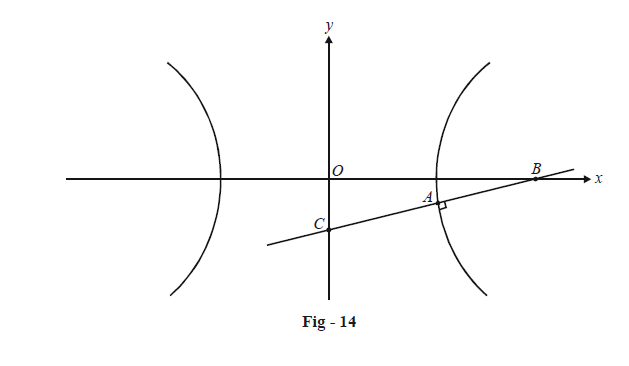# Examples on Normals to Hyperbolas Set 2

Go back to  'Hyperbola'

Example - 19

The normal at a point A on a hyperbola of eccentricity e intersects its transverse and conjugate axes at B and C respectively, as shown in Fig - 14. Show that the locus of the mid-point of BC is a hyperbola of eccentricity \begin{align}\frac{e}{{\sqrt {{e^2} - 1} }}.\end{align}Solution : Let the point A be $$(a\sec \theta ,\,b\tan \theta ).$$ Then the equation for the normal at A is

$ax\sin \theta + by = ({a^2} + {b^2})\tan \theta$

The points B and C can easily be determined using this equation:

\begin{align}& \text{Point }\quad \text{B :}\qquad Put\,\,y=0\qquad \Rightarrow \qquad x=\frac{({{a}^{2}}+{{b}^{2}})}{a\cos \theta } \\ & \qquad \qquad \qquad \qquad \qquad \quad \qquad\; \Rightarrow \qquad B\equiv \left( \frac{{{a}^{2}}+{{b}^{2}}}{a\cos \theta },\,\,0 \right) \\ & \text{Point }\quad \text{C:}\qquad \text{Put }x=0\qquad \Rightarrow \qquad y=\frac{({{a}^{2}}+{{b}^{2}})\tan \theta }{b} \\ & \qquad \qquad \qquad \qquad \qquad \qquad\;\;\; \Rightarrow \qquad C\equiv \left( 0,\,\,\frac{({{a}^{2}}+{{b}^{2}})\tan \theta }{b} \right) \end{align}

Let the mid point of BC be  $$P(h,\,\,k),$$ whose locus we wish to determine. We have,

\begin{align}&\qquad\;\;\; h = \frac{{({a^2} + {b^2})}}{{2a\cos \theta }},\,\,\,\,\,k = \frac{{({a^2} + {b^2})\tan \theta }}{{2b}} \\\\ &\Rightarrow \quad \sec \theta = \frac{{2ah}}{{{a^2} + {b^2}}},\,\,\,\,\,\tan \theta = \frac{{2bk}}{{{a^2} + {b^2}}} \\ \end{align}

Eliminating $$\theta$$ using the relation  $${\sec ^2}\theta - {\tan ^2}\theta = 1,$$ we have

$\frac{{4{a^2}{h^2}}}{{{{({a^2} + {b^2})}^2}}} - \frac{{4{b^2}{k^2}}}{{{{({a^2} + {b^2})}^2}}} = 1$

Thus, the locus of P is

\begin{align}&\frac{{{x^2}}}{{{{\left( {\begin{align}\frac{{{a^2} + {b^2}}}{{2a}}\end{align}} \right)}^2}}} - \frac{{{y^2}}}{{{{\left( {\begin{align}\frac{{{a^2} + {b^2}}}{{2b}}\end{align}} \right)}^2}}} = 1\end{align}

This is obviously a hyperbola with eccentricity E given by

\begin{align} &\quad\quad\; {{E}^{2}}=1+\frac{{{\left( \begin{align}\frac{{{a}^{2}}+{{b}^{2}}}{2b}\end{align} \right)}^{2}}}{{{\left( \begin{align}\frac{{{a}^{2}}+{{b}^{2}}}{2a}\end{align} \right)}^{2}}} \\ & \qquad\quad\;=1+\frac{{{a}^{2}}}{{{b}^{2}}} \\ & \qquad\quad\;=1+\frac{1}{{}^{{{b}^{2}}}\!\!\diagup\!\!{}_{{{a}^{2}}}\;} \\ & \qquad\quad\;=1+\frac{1}{1+\begin{align}\frac{{{b}^{2}}}{{{a}^{2}}}\end{align}-1} \\ & \qquad\quad\;=1+\frac{1}{{{e}^{2}}-1}\,\,\,\,\,\,\,\,\,\,\,\,\left( \because \,\,\,\,\,{{e}^{2}}=1+\frac{{{b}^{2}}}{{{a}^{2}}} \right) \\ & \qquad\quad\;=\frac{{{e}^{2}}}{{{e}^{2}}-1} \\ & \Rightarrow\quad E=\frac{e}{\sqrt{{{e}^{2}}-1}} \end{align}

As asserted, this is the eccentricity of the hyperbola described by P.

Example - 20

Find the locus of the foot of the perpendicular drawn from the center C of the hyperbola  \begin{align}\frac{{{x}^{2}}}{{{a}^{2}}}-\frac{{{y}^{2}}}{{{b}^{2}}}=1\end{align} on any normal.

Solution : Any normal to the given hyperbola has the form

$ax\cos \theta +by\cot \theta ={{a}^{2}}+{{b}^{2}}$

Let the foot of the perpendicular from C(0, 0) to this normal be $$P(h,\,\,k).$$  We have

\begin{align} & \,\,\,\,\,\,\,\,\,\,\,\,\,\,\,\,\,\,\,\,\,\,\,\,\,\,\,ah\cos \theta +bk\cot \theta ={{a}^{2}}+{{b}^{2}}\,\,\,\,\,\,\,\,\,\,\,\,\,\,\,\,\,\,\,\,\ldots \left( 1 \right) \\\\ & \text{and}\,\,\,\,\,\,\,\,\,\,\,\,\,\,\,\,\,\,\,\,\,\frac{k}{h}\times \frac{-a\cos \theta }{b\cot \theta }=-1\,\,\,\,\,\,\,\,\,\,\,\,\,\,\,\,\,\,\,\,\,\,\,\,\,\,\,\,\,\,\,\,\,\,\,\,\,\ldots \left( 2 \right)\,\,\, \\\\ & \left( CP\bot (\text{the normal)} \right) \\ \end{align}

We can easily eliminate $$\theta$$  from these two relations; from (2),

\begin{align} &\;\;\;\;\;\ \sin \theta =\frac{bh}{ak} \\ & \Rightarrow \cos \theta =\sqrt{1-\frac{{{b}^{2}}{{h}^{2}}}{{{a}^{2}}{{k}^{2}}}};\cot \theta =\frac{\sqrt{{{a}^{2}}{{k}^{2}}-{{b}^{2}}{{h}^{2}}}}{bh}\,\,\,\,\,\,\,\,\,\,\,\,\,\,\,\,\,\,\,\,\ldots \left( 3 \right) \\ \end{align}

Using (3) in (2), we have

\begin{align} &\;\;\;\;\;\; ah\sqrt{1-\frac{{{b}^{2}}{{h}^{2}}}{{{a}^{2}}{{k}^{2}}}}+bk\frac{\sqrt{{{a}^{2}}{{k}^{2}}-{{b}^{2}}{{h}^{2}}}}{bh}={{a}^{2}}+{{b}^{2}} \\\\ & \Rightarrow \left( \frac{h}{k}+\frac{k}{h} \right)\sqrt{{{a}^{2}}{{k}^{2}}-{{b}^{2}}{{h}^{2}}}={{a}^{2}}+{{b}^{2}} \\\\ & \Rightarrow {{({{h}^{2}}+{{k}^{2}})}^{2}}({{a}^{2}}{{k}^{2}}-{{b}^{2}}{{h}^{2}})={{h}^{2}}{{k}^{2}}({{a}^{2}}+{{b}^{2}}) \\ \end{align}

Thus, the locus of P is

${{({{x}^{2}}+{{y}^{2}})}^{2}}({{a}^{2}}{{y}^{2}}-{{b}^{2}}{{x}^{2}})={{x}^{2}}{{y}^{2}}({{a}^{2}}+{{b}^{2}})$

## TRY YOURSELF - III

Q1. Show that the feet of the normals drawn from a point  $$P(h,\,\,k)$$ to the hyperbola \begin{align}\frac{{{x}^{2}}}{{{a}^{2}}}-\frac{{{y}^{2}}}{{{b}^{2}}}=1\end{align} lie on the curve

${{a}^{2}}y(x-h)+{{b}^{2}}x(y-k)=0.$

Q2. Let the normal at any point P on the hyperbola  \begin{align}\frac{{{x}^{2}}}{{{a}^{2}}}-\frac{{{y}^{2}}}{{{b}^{2}}}=1\end{align} meet the transverse and the conjugate axis in A and B respectively. From O, the center of the hyperbola, perpendicular OQ is dropped onto the normal at P. Show that

$$PQ\cdot PA={{b}^{2}}$$ and $$PQ\cdot PB={{a}^{2}}$$